Linear Functions

LEARNING OBJECTIVES

By the end of this lesson, you will be able to:

• Represent a linear function.
• Determine whether a linear function is increasing, decreasing, or constant.
• Calculate and interpret slope.
• Write the point-slope form of an equation.
• Write and interpret a linear function.
Introduction to Linear Functions

A bamboo forest in China (credit: “JFXie”/Flickr)Imagine placing a plant in the ground one day and finding that it has doubled its height just a few days later. Although it may seem incredible, this can happen with certain types of bamboo species. These members of the grass family are the fastest-growing plants in the world. One species of bamboo has been observed to grow nearly 1.5 inches every hour.1 In a twenty-four hour period, this bamboo plant grows about 36 inches, or an incredible 3 feet! A constant rate of change, such as the growth cycle of this bamboo plant, is a linear function.

Recall from Functions and Function Notation that a function is a relation that assigns to every element in the domain exactly one element in the range. Linear functions are a specific type of function that can be used to model many real-world applications, such as plant growth over time. In this chapter, we will explore linear functions, their graphs, and how to relate them to data.

Footnotes

1. 1 http://www.guinnessworldrecords.com/records-3000/fastest-growing-plant/

Just as with the growth of a bamboo plant, there are many situations that involve constant change over time. Consider, for example, the first commercial maglev train in the world, the Shanghai MagLev Train. It carries passengers comfortably for a 30-kilometer trip from the airport to the subway station in only eight minutes.

Suppose a maglev train were to travel a long distance, and that the train maintains a constant speed of 83 meters per second for a period of time once it is 250 meters from the station. How can we analyze the train’s distance from the station as a function of time? In this section, we will investigate a kind of function that is useful for this purpose, and use it to investigate real-world situations such as the train’s distance from the station at a given point in time.

Representing Linear Functions

The function describing the train’s motion is a linear function, which is defined as a function with a constant rate of change, that is, a polynomial of degree 1. There are several ways to represent a linear function, including word form, function notation, tabular form, and graphical form. We will describe the train’s motion as a function using each method.

Representing a Linear Function in Word Form

Let’s begin by describing the linear function in words. For the train problem we just considered, the following word sentence may be used to describe the function relationship.

• The train’s distance from the station is a function of the time during which the train moves at a constant speed plus its original distance from the station when it began moving at constant speed.

The speed is the rate of change. Recall that a rate of change is a measure of how quickly the dependent variable changes with respect to the independent variable. The rate of change for this example is constant, which means that it is the same for each input value. As the time (input) increases by 1 second, the corresponding distance (output) increases by 83 meters. The train began moving at this constant speed at a distance of 250 meters from the station.

Representing a Linear Function in Function Notation

Another approach to representing linear functions is by using function notation. One example of function notation is an equation written in the form known as the slope-intercept form of a line, where $x$ is the input value, $m$ is the rate of change, and $b$ is the initial value of the dependent variable.

$\begin{cases}\text{Equation form}\hfill & y=mx+b\hfill \\ \text{Equation notation}\hfill & f\left(x\right)=mx+b\hfill \end{cases}$

In the example of the train, we might use the notation $D\left(t\right)$ in which the total distance $D$
is a function of the time $t$. The rate, $m$, is 83 meters per second. The initial value of the dependent variable $b$ is the original distance from the station, 250 meters. We can write a generalized equation to represent the motion of the train.

$D\left(t\right)=83t+250$

Representing a Linear Function in Tabular Form

A third method of representing a linear function is through the use of a table. The relationship between the distance from the station and the time is represented in the table in Figure 1. From the table, we can see that the distance changes by 83 meters for every 1 second increase in time.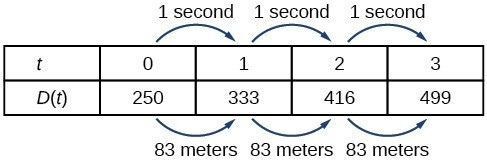Figure 1. Tabular representation of the function D showing selected input and output values

Q & A

Can the input in the previous example be any real number?

No. The input represents time, so while nonnegative rational and irrational numbers are possible, negative real numbers are not possible for this example. The input consists of non-negative real numbers.

Representing a Linear Function in Graphical Form

Another way to represent linear functions is visually, using a graph. We can use the function relationship from above, $D\left(t\right)=83t+250$, to draw a graph, represented in the graph in Figure 2. Notice the graph is a line. When we plot a linear function, the graph is always a line.

The rate of change, which is constant, determines the slant, or slope of the line. The point at which the input value is zero is the vertical intercept, or y-intercept, of the line. We can see from the graph that the y-intercept in the train example we just saw is $\left(0,250\right)$ and represents the distance of the train from the station when it began moving at a constant speed.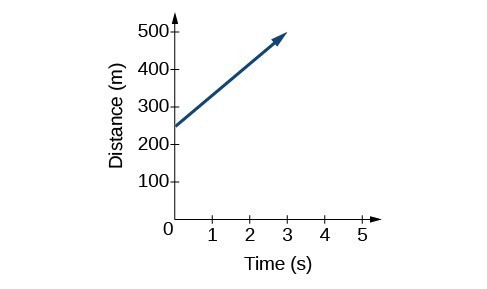Figure 2. The graph of $D\left(t\right)=83t+250$. Graphs of linear functions are lines because the rate of change is constant.

Notice that the graph of the train example is restricted, but this is not always the case. Consider the graph of the line $f\left(x\right)=2{x}_{}+1$. Ask yourself what numbers can be input to the function, that is, what is the domain of the function? The domain is comprised of all real numbers because any number may be doubled, and then have one added to the product.

A General Note: Linear Function

A linear function is a function whose graph is a line. Linear functions can be written in the slope-intercept form of a line

$f\left(x\right)=mx+b$

where $b$ is the initial or starting value of the function (when input, $x=0$), and $m$ is the constant rate of change, or slope of the function. The y-intercept is at $\left(0,b\right)$.

Example 1: Using a Linear Function to Find the Pressure on a Diver

The pressure, $P$, in pounds per square inch (PSI) on the diver in Figure 3 depends upon her depth below the water surface, $d$, in feet. This relationship may be modeled by the equation, $P\left(d\right)=0.434d+14.696$. Restate this function in words.Figure 3. (credit: Ilse Reijs and Jan-Noud Hutten)

Solution

To restate the function in words, we need to describe each part of the equation. The pressure as a function of depth equals four hundred thirty-four thousandths times depth plus fourteen and six hundred ninety-six thousandths.

Analysis of the Solution

The initial value, 14.696, is the pressure in PSI on the diver at a depth of 0 feet, which is the surface of the water. The rate of change, or slope, is 0.434 PSI per foot. This tells us that the pressure on the diver increases 0.434 PSI for each foot her depth increases.

Determining Whether a Linear Function is Increasing, Decreasing, or Constant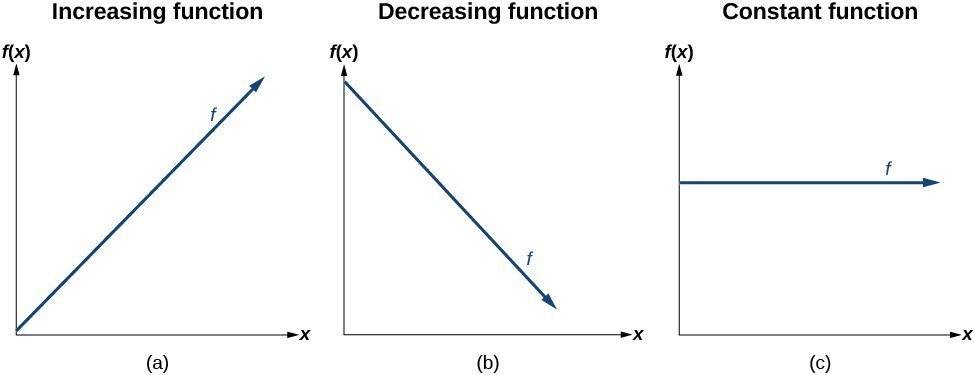Figure 4

The linear functions we used in the two previous examples increased over time, but not every linear function does. A linear function may be increasing, decreasing, or constant.

For an increasing function, as with the train example,

the output values increase as the input values increase.

The graph of an increasing function has a positive slope. A line with a positive slope slants upward from left to right as in (a).

For a decreasing function, the slope is negative.

The output values decrease as the input values increase.

A line with a negative slope slants downward from left to right as in (b). If the function is constant, the output values are the same for all input values so the slope is zero. A line with a slope of zero is horizontal as in (c).

A General Note: Increasing and Decreasing Functions

The slope determines if the function is an increasing linear function, a decreasing linear function, or a constant function.

• $f\left(x\right)=mx+b\text{ is an increasing function if }m>0$.
• $f\left(x\right)=mx+b\text{ is an decreasing function if }m<0$.
• $f\left(x\right)=mx+b\text{ is a constant function if }m=0$.

Example 2: Deciding whether a Function Is Increasing, Decreasing, or Constant

Some recent studies suggest that a teenager sends an average of 60 texts per day. For each of the following scenarios, find the linear function that describes the relationship between the input value and the output value. Then, determine whether the graph of the function is increasing, decreasing, or constant.

1. The total number of texts a teen sends is considered a function of time in days. The input is the number of days, and output is the total number of texts sent.
2. A teen has a limit of 500 texts per month in his or her data plan. The input is the number of days, and output is the total number of texts remaining for the month.

Example 9: Writing an Equation for a Linear Function Given Two Points

If f is a linear function, with $f\left(3\right)=-2$ , and $f\left(8\right)=1$, find an equation for the function in slope-intercept form.

Solution

We can write the given points using coordinates.

$\begin{cases}f\left(3\right)=-2\to \left(3,-2\right)\hfill \\ f\left(8\right)=1\to \left(8,1\right)\hfill \end{cases}$

We can then use the points to calculate the slope.

$\begin{cases} m=\frac{{y}_{2}-{y}_{1}}{{x}_{2}-{x}_{1}}\hfill \\ \text{ }=\frac{1-\left(-2\right)}{8 - 3}\hfill \\ \text{ }=\frac{3}{5}\hfill \end{cases}$

Substitute the slope and the coordinates of one of the points into the point-slope form.

$\begin{cases}\text{ }y-{y}_{1}=m\left(x-{x}_{1}\right)\hfill \\ y-\left(-2\right)=\frac{3}{5}\left(x - 3\right)\hfill \end{cases}$

We can use algebra to rewrite the equation in the slope-intercept form.

$\begin{cases}y+2=\frac{3}{5}\left(x - 3\right)\hfill \\ y+2=\frac{3}{5}x-\frac{9}{5}\hfill \\ \text{ }y=\frac{3}{5}x-\frac{19}{5}\hfill \end{cases}$

Try It 5

If $f\left(x\right)$ is a linear function, with $f\left(2\right)=-11$, and $f\left(4\right)=-25$, find an equation for the function in slope-intercept form.

Solution

Modeling Real-World Problems with Linear Functions

In the real world, problems are not always explicitly stated in terms of a function or represented with a graph. Fortunately, we can analyze the problem by first representing it as a linear function and then interpreting the components of the function. As long as we know, or can figure out, the initial value and the rate of change of a linear function, we can solve many different kinds of real-world problems.

How To: Given a linear function f and the initial value and rate of change, evaluate f(c).

1. Determine the initial value and the rate of change (slope).
2. Substitute the values into $f\left(x\right)=mx+b$.
3. Evaluate the function at $x=c$.

Example 10: Using a Linear Function to Determine the Number of Songs in a Music Collection

Marcus currently has 200 songs in his music collection. Every month, he adds 15 new songs. Write a formula for the number of songs, N, in his collection as a function of time, t, the number of months. How many songs will he own in a year?

Solution

The initial value for this function is 200 because he currently owns 200 songs, so N(0) = 200, which means that b = 200.Figure 12

The number of songs increases by 15 songs per month, so the rate of change is 15 songs per month. Therefore we know that m = 15. We can substitute the initial value and the rate of change into the slope-intercept form of a line.

We can write the formula $N\left(t\right)=15t+200$.

With this formula, we can then predict how many songs Marcus will have in 1 year (12 months). In other words, we can evaluate the function at t = 12.

$\begin{cases}N\left(12\right)=15\left(12\right)+200\hfill \\ \text{ }=180+200\hfill \\ \text{ }=380\hfill \end{cases}$

Marcus will have 380 songs in 12 months.

Analysis of the Solution

Notice that N is an increasing linear function. As the input (the number of months) increases, the output (number of songs) increases as well.

Example 11: Using a Linear Function to Calculate Salary Plus Commission

Working as an insurance salesperson, Ilya earns a base salary plus a commission on each new policy. Therefore, Ilya’s weekly income, I, depends on the number of new policies, n, he sells during the week. Last week he sold 3 new policies, and earned $760 for the week. The week before, he sold 5 new policies and earned$920. Find an equation for I(n), and interpret the meaning of the components of the equation.

Solution

The given information gives us two input-output pairs: (3, 760) and (5, 920). We start by finding the rate of change.

$\begin{cases}m=\frac{920 - 760}{5 - 3}\hfill \\ \text{ }=\frac{160}{\text{2 policies}}\hfill \\ \text{ }=80\text{ per policy}\hfill \end{cases}$

Keeping track of units can help us interpret this quantity. Income increased by $160 when the number of policies increased by 2, so the rate of change is$80 per policy. Therefore, Ilya earns a commission of $80 for each policy sold during the week. We can then solve for the initial value. $\begin{cases}\text{ }I\left(n\right)=80n+b\hfill & \hfill \\ \text{ }760=80\left(3\right)+b\hfill & \text{when }n=3, I\left(3\right)=760\hfill \\ 760 - 80\left(3\right)=b\hfill & \hfill \\ \text{ }520=b\hfill & \hfill \end{cases}$ The value of b is the starting value for the function and represents Ilya’s income when n = 0, or when no new policies are sold. We can interpret this as Ilya’s base salary for the week, which does not depend upon the number of policies sold. We can now write the final equation. $I\left(n\right)=80n+520$ Our final interpretation is that Ilya’s base salary is$520 per week and he earns an additional $80 commission for each policy sold. Example 12: Using Tabular Form to Write an Equation for a Linear Function The table below relates the number of rats in a population to time, in weeks. Use the table to write a linear equation.  w, number of weeks 0 2 4 6 P(w), number of rats 1000 1080 1160 1240 Solution We can see from the table that the initial value for the number of rats is 1000, so b = 1000. Rather than solving for m, we can tell from looking at the table that the population increases by 80 for every 2 weeks that pass. This means that the rate of change is 80 rats per 2 weeks, which can be simplified to 40 rats per week. $P\left(w\right)=40w+1000$ If we did not notice the rate of change from the table we could still solve for the slope using any two points from the table. For example, using (2, 1080) and (6, 1240) $\begin{cases}m=\frac{1240 - 1080}{6 - 2}\hfill \\ \text{ }=\frac{160}{4}\hfill \\ \text{ }=40\hfill \end{cases}$ Q & A Is the initial value always provided in a table of values like the table in Example 12? No. Sometimes the initial value is provided in a table of values, but sometimes it is not. If you see an input of 0, then the initial value would be the corresponding output. If the initial value is not provided because there is no value of input on the table equal to 0, find the slope, substitute one coordinate pair and the slope into $f\left(x\right)=mx+b$, and solve for b. Try It 6 A new plant food was introduced to a young tree to test its effect on the height of the tree. The table below shows the height of the tree, in feet, x months since the measurements began. Write a linear function, H(x), where x is the number of months since the start of the experiment.  x 0 2 4 8 12 H(x) 12.5 13.5 14.5 16.5 18.5 Solution Key Equations  slope-intercept form of a line $f\left(x\right)=mx+b$ slope $m=\frac{\text{change in output (rise)}}{\text{change in input (run)}}=\frac{\Delta y}{\Delta x}=\frac{{y}_{2}-{y}_{1}}{{x}_{2}-{x}_{1}}$ point-slope form of a line $y-{y}_{1}=m\left(x-{x}_{1}\right)$ Key Concepts • The ordered pairs given by a linear function represent points on a line. • Linear functions can be represented in words, function notation, tabular form, and graphical form. • The rate of change of a linear function is also known as the slope. • An equation in the slope-intercept form of a line includes the slope and the initial value of the function. • The initial value, or y-intercept, is the output value when the input of a linear function is zero. It is the y-value of the point at which the line crosses the y-axis. • An increasing linear function results in a graph that slants upward from left to right and has a positive slope. • A decreasing linear function results in a graph that slants downward from left to right and has a negative slope. • A constant linear function results in a graph that is a horizontal line. • Analyzing the slope within the context of a problem indicates whether a linear function is increasing, decreasing, or constant. • The slope of a linear function can be calculated by dividing the difference between y-values by the difference in corresponding x-values of any two points on the line. • The slope and initial value can be determined given a graph or any two points on the line. • One type of function notation is the slope-intercept form of an equation. • The point-slope form is useful for finding a linear equation when given the slope of a line and one point. • The point-slope form is also convenient for finding a linear equation when given two points through which a line passes. • The equation for a linear function can be written if the slope m and initial value are known. • A linear function can be used to solve real-world problems. • A linear function can be written from tabular form. Glossary decreasing linear function a function with a negative slope: If $f\left(x\right)=mx+b, \text{then} m<0$. increasing linear function a function with a positive slope: If $f\left(x\right)=mx+b, \text{then} m>0$. linear function a function with a constant rate of change that is a polynomial of degree 1, and whose graph is a straight line point-slope form the equation for a line that represents a linear function of the form $y-{y}_{1}=m\left(x-{x}_{1}\right)$ slope the ratio of the change in output values to the change in input values; a measure of the steepness of a line slope-intercept form the equation for a line that represents a linear function in the form $f\left(x\right)=mx+b$ y-intercept the value of a function when the input value is zero; also known as initial value Problem Set 1. Terry is skiing down a steep hill. Terry’s elevation, E(t), in feet after t seconds is given by $E\left(t\right)=3000-70t$. Write a complete sentence describing Terry’s starting elevation and how it is changing over time. 2. Maria is climbing a mountain. Maria’s elevation, E(t), in feet after t minutes is given by $E\left(t\right)=1200+40t$. Write a complete sentence describing Maria’s starting elevation and how it is changing over time. 3. Jessica is walking home from a friend’s house. After 2 minutes she is 1.4 miles from home. Twelve minutes after leaving, she is 0.9 miles from home. What is her rate in miles per hour? 4. Sonya is currently 10 miles from home and is walking farther away at 2 miles per hour. Write an equation for her distance from home t hours from now. 5. A boat is 100 miles away from the marina, sailing directly toward it at 10 miles per hour. Write an equation for the distance of the boat from the marina after t hours. 6. Timmy goes to the fair with$40. Each ride costs $2. How much money will he have left after riding $n$ rides? For the following exercises, determine whether the equation of the curve can be written as a linear function. 7. $y=\frac{1}{4}x+6$ 8. $y=3x - 5$ 9. $y=3{x}^{2}-2$ 10. $3x+5y=15$ 11. $3{x}^{2}+5y=15$ 12. $3x+5{y}^{2}=15$ 13. $-2{x}^{2}+3{y}^{2}=6$ 14. $-\frac{x - 3}{5}=2y$ For the following exercises, determine whether each function is increasing or decreasing. 15. $f\left(x\right)=4x+3$ 16. $g\left(x\right)=5x+6$ 17. $a\left(x\right)=5 - 2x$ 18. $b\left(x\right)=8 - 3x$ 19. $h\left(x\right)=-2x+4$ 20. $k\left(x\right)=-4x+1$ 21. $j\left(x\right)=\frac{1}{2}x - 3$ 22. $p\left(x\right)=\frac{1}{4}x - 5$ 23. $n\left(x\right)=-\frac{1}{3}x - 2$ 24. $m\left(x\right)=-\frac{3}{8}x+3$ For the following exercises, find the slope of the line that passes through the two given points. 25. $\left(2,\text{ }4\right)$ and $\left(4,\text{ 10}\right)$ 26. $\left(1,\text{ 5}\right)$ and $\left(4,\text{ 11}\right)$ 27. $\left(-1,\text{4}\right)$ and $\left(5,\text{2}\right)$ 28. $\left(8,-2\right)$ and $\left(4,6\right)$ 29. $\left(6,\text{ }11\right)$ and $\left(-4, 3\right)$ For the following exercises, given each set of information, find a linear equation satisfying the conditions, if possible. 30. $f\left(-5\right)=-4$, and $f\left(5\right)=2$ 31. $f\left(-1\right)=4$ and $f\left(5\right)=1$ 32. $\left(2,4\right)$ and $\left(4,10\right)$ 33. Passes through $\left(1,5\right)$ and $\left(4,11\right)$ 34. Passes through $\left(-1,\text{ 4}\right)$ and $\left(5,\text{ 2}\right)$ 35. Passes through $\left(-2,\text{ 8}\right)$ and $\left(4,\text{ 6}\right)$ 36. x intercept at $\left(-2,\text{ 0}\right)$ and y intercept at $\left(0,-3\right)$ 37. x intercept at $\left(-5,\text{ 0}\right)$ and y intercept at $\left(0,\text{ 4}\right)$ For the following exercises, find the slope of the lines graphed. 38.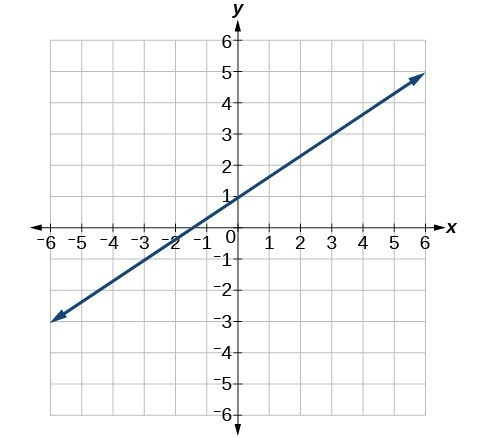39.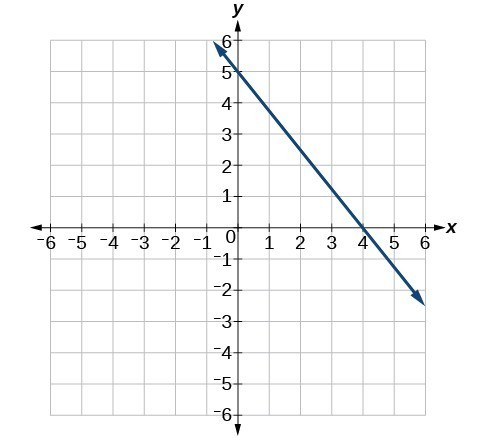40.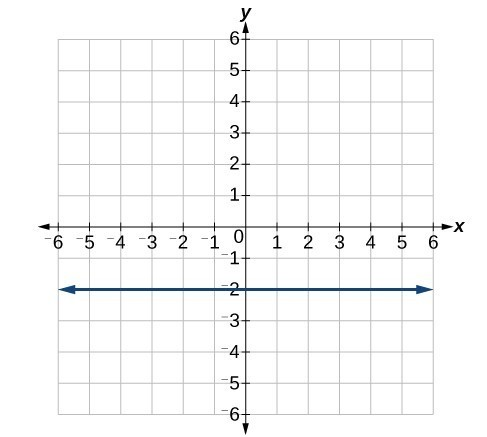For the following exercises, write an equation for the lines graphed. 41.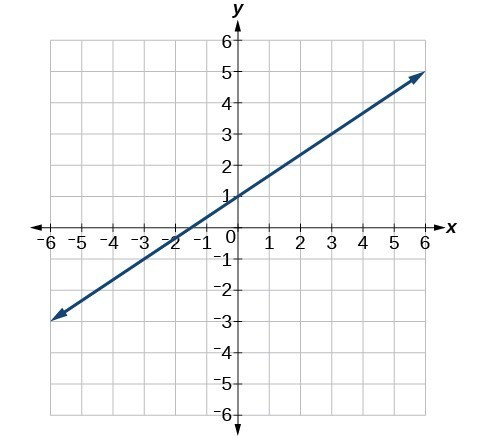42.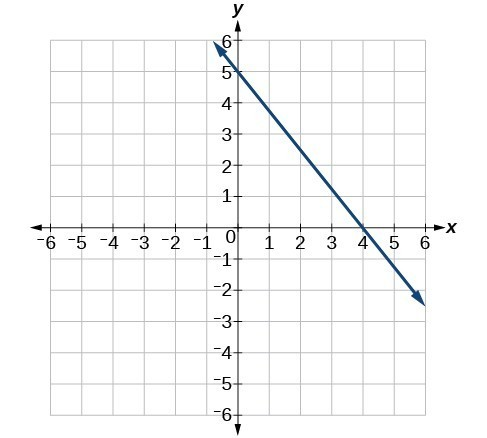43.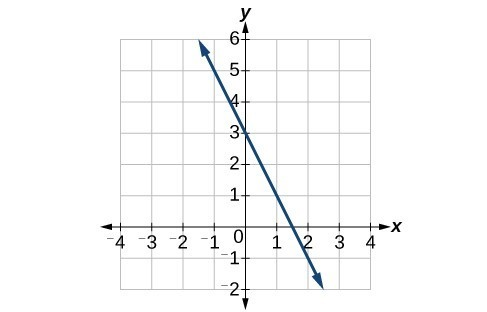44.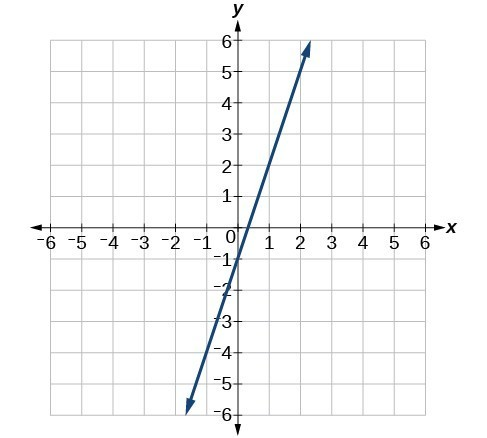45.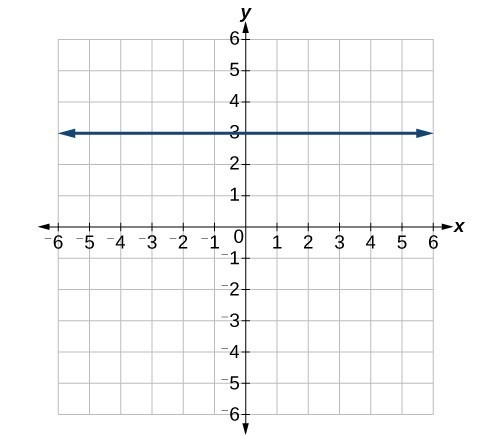46.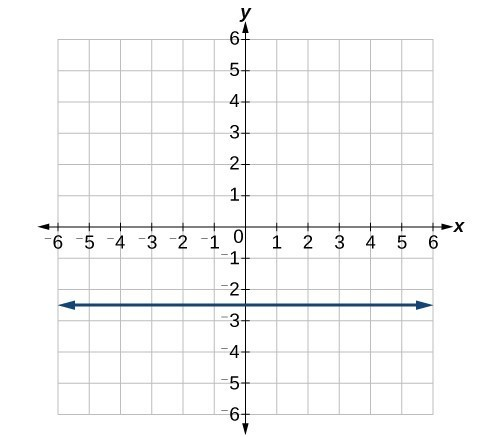For the following exercises, which of the tables could represent a linear function? For each that could be linear, find a linear equation that models the data. 47.  x 0 5 10 15 g(x) 5 –10 –25 –40 48.  x 0 5 10 15 h(x) 5 30 105 230 49.  x 0 5 10 15 f(x) –5 20 45 70 50.  x 5 10 20 25 k(x) 28 13 58 73 51.  x 0 2 4 6 g(x) 6 –19 –44 –69 52.  x 2 4 6 8 f(x) –4 16 36 56 53.  x 2 4 6 8 f(x) –4 16 36 56 54.  x 0 2 6 8 k(x) 6 31 106 231 55. If is a linear function, $f\left(0.1\right)=11.5, \text{ and} f\left(0.4\right)=-5.9$, find an equation for the function. 56. Graph the function f on a domain of $\left[-10, 10\right]: f\left(x\right)=0.02x - 0.01$. Enter the function in a graphing utility. For the viewing window, set the minimum value of to be –10 and the maximum value of x to be 10. 57. Graph the function f on a domain of $\left[-10, 10\right]:f\left(x\right)=2,500x+4,000$ 58. The table below shows the input, w, and output, k, for a linear function k. a. Fill in the missing values of the table. b. Write the linear function k, round to 3 decimal places.  w –10 5.5 67.5 b k 30 –26 a –44 59. shows the input, p, and output, q, for a linear function q. a. Fill in the missing values of the table. b. Write the linear function k.  p 0.5 0.8 12 b q 400 700 a 1,000,000 60. Graph the linear function f on a domain of [–10, 10] for the function whose slope is $\frac{1}{8}$ and y-intercept is $\frac{31}{16}$. Label the points for the input values of –10 and 10. 61. Graph the linear function f on a domain of [–0.1, 0.1] for the function whose slope is 75 and y-intercept is –22.5. Label the points for the input values of –0.1 and 0.1. 62. Graph the linear function f where $f\left(x\right)=ax+b$ on the same set of axes on a domain of [–4, 4] for the following values of a and b. $a=2;\text{ }b=3$ $a=2;\text{ }b=4$ $a=2;\text{ }b=-4$ $a=2;\text{ }b=-5$ 63. Find the value of x if a linear function goes through the following points and has the following slope: $\left(x,2\right),\left(-4,6\right),m=3$ 64. Find the value of y if a linear function goes through the following points and has the following slope: $\left(10,y\right),\left(25,100\right),m=-5$ 65. Find the equation of the line that passes through the following points: $\left(a,\text{ }b\right)$ and $\left(a,\text{ }b+1\right)$ 66. Find the equation of the line that passes through the following points: $\left(2a,\text{ }b\right)$ and $\left(a,\text{ }b+1\right)$ 67. Find the equation of the line that passes through the following points: $\left(a,\text{ }0\right)$ and $\left(c,\text{ }d\right)$ 68. At noon, a barista notices that she has$20 in her tip jar. If she makes an average of $0.50 from each customer, how much will she have in her tip jar if she serves n more customers during her shift? 69. A gym membership with two personal training sessions costs$125, while gym membership with five personal training sessions costs $260. What is cost per session? 70. A clothing business finds there is a linear relationship between the number of shirts, n, it can sell and the price, p, it can charge per shirt. In particular, historical data shows that 1,000 shirts can be sold at a price of$30, while 3,000 shirts can be sold at a price of $22. Find a linear equation in the form $p\left(n\right)=mn+b$ that gives the price p they can charge for n shirts. 71. A phone company charges for service according to the formula: $C\left(n\right)=24+0.1n$, where n is the number of minutes talked, and $C\left(n\right)$ is the monthly charge, in dollars. Find and interpret the rate of change and initial value. 72. A farmer finds there is a linear relationship between the number of bean stalks, n, she plants and the yield, y, each plant produces. When she plants 30 stalks, each plant yields 30 oz of beans. When she plants 34 stalks, each plant produces 28 oz of beans. Find a linear relationships in the form $y=\mathrm{mn}+b$ that gives the yield when n stalks are planted. 73. A city’s population in the year 1960 was 287,500. In 1989 the population was 275,900. Compute the rate of growth of the population and make a statement about the population rate of change in people per year. 74. A town’s population has been growing linearly. In 2003, the population was 45,000, and the population has been growing by 1,700 people each year. Write an equation, $P\left(t\right)$, for the population t years after 2003. 75. Suppose that average annual income (in dollars) for the years 1990 through 1999 is given by the linear function: $I\left(x\right)=1054x+23,286$, where x is the number of years after 1990. Which of the following interprets the slope in the context of the problem? a. As of 1990, average annual income was$23,286.
b. In the ten-year period from 1990–1999, average annual income increased by a total of $1,054. c. Each year in the decade of the 1990s, average annual income increased by$1,054.
d. Average annual income rose to a level of \$23,286 by the end of 1999.

76. When temperature is 0 degrees Celsius, the Fahrenheit temperature is 32. When the Celsius temperature is 100, the corresponding Fahrenheit temperature is 212. Express the Fahrenheit temperature as a linear function of C, the Celsius temperature, $F\left(C\right)$.

a. Find the rate of change of Fahrenheit temperature for each unit change temperature of Celsius.
b. Find and interpret $F\left(28\right)$.
c. Find and interpret $F\left(-40\right)$.